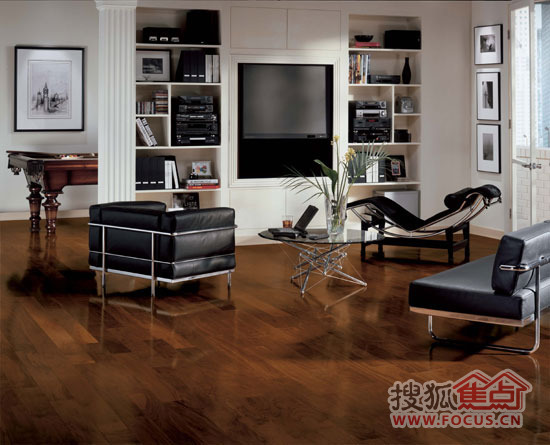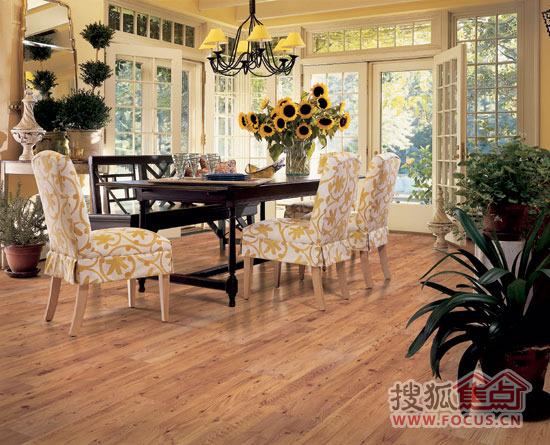|

# 阿姆斯壮莊民亮:150年历程 美式经典地板的辉煌2010年3月23日，作为亚洲最大的地面材料展会，第十二届中国国际地面材料及铺装技术展览会在新国际博览中心隆重举行。虽然天空不作美，但正如某位参展商在交谈中向我们调侃的，虽然外面阴雨绵绵，但馆内的温度却有120°C之高，可见本届展会吸引观众人流之多。据悉，本次展会为期三天，搜狐家居作为展会网络支持媒体，也将在这三天内对展会盛况、现场活动进行全程报道，并对重点参展企业进行主题访谈——

【主持人】：莊总，您好！非常高兴今天能够邀请到您接受搜狐家居的采访。今年我们的展位给我的第一个感觉就是非常大，好像是有420平，很开阔的感觉，这样一个整体风格是不是同我们倡导的美式风格有关？另外我一进门就看到，我们阿姆斯壮150周年庆的球体标识门楣，那么不知道在这样一个值得纪念的日子，我们会给我们的观众、我们的买家展示什么样的产品？【莊民亮】：好的。我们阿姆斯壮，一开始在国内，是在工装这方面比较多，但随着我们在工程上的日渐成熟，现在我们也逐步跨入家用地板的领域。可以说，现在我们是作为商用与家用地板的全球领导品牌，来为消费者提供最全面的地面材料解决方案。又因为这几年，美式家居风格逐步进入中国，那么传统意义上，我们感受到的美式家居风格是以大气、舒适、温暖、朴实、典雅为主，所以在家装过程中，地板选择也由于会影响甚至决定整体家装风格开始受到更多的重视。所以这次展会，我们阿姆斯壮的展位主要带来四种不同风格的美式地板，提供给消费者有关美式生活的不同选择。首先是新古典主义风格。这个展区是以现代手法和材质还原古典气质，将之以更简洁的线条来表现，呈现古典与现代的双重审美效果。我们的樱桃木就是典型的新古典主义风格产品，其中粉红樱桃和法国吐司两款都十分经典。尤其是粉红樱桃产品精选了美国樱桃木面皮，使用晶面立方技术，比一般平面产品更有质感。其次是美式现代风格展区，这个展区着重简单清晰的线条，以色调清新的加拿大枫木自然色配合现代感的家具摆设，呈现简洁而富自由感的现代气质。因为加拿大枫木的色泽纹理均匀而不乏细腻，是槭树科中公认纹路最漂亮，密度最大，具有最好加工性能的木种。第三个，是我们的美式古典风格展区，这个展区以复古感觉，带来怀旧经典气息。那么这个展区主推的就是我们的山核桃木产品。其中，低调而不失浪漫的迷人琥珀款颜色厚重，具有复古又不失温馨舒适的气息，可以说是搭配美式古典家居的最佳选择。第四个是美式田园风格展区，这个展区是一些比较爱好自由的消费者特别喜欢的，因为你可以在这个展区感觉到扑面而来的惬意质朴的情调，我们的胡桃色楸木独具层次感的天然木纹，配搭田园风格的布艺家居，别致清新，宁静雅致。

【主持人】：那么在您看来，我们这四种风格的地板目前在消费群中最受欢迎的是哪一种？

【莊民亮】：这个也比较难做定论。因为其实，每一种风格都有他独特的味道，独特的理念在其中，那么其实我们的消费群体跨度也很大，所以每种地板都有比较稳定的拥护者或者说消费群。比如说你崇尚自然朴素，讲求天然、随意、简单，那么乡村田园风格应该说是更适合你的，比如说你是比较稳重的那种性格，那么美式古典风格就比较适合你。所以其实在这方面我们也希望提供给消费者更多的选择空间。

【主持人】：您刚才提到说提供给消费者更多的选择空间，好像我们在设计师这一块也一直非常活跃，经常会有相关的设计师活动，而不少消费者也比较希望能够通过设计师来为他们提供有关生活理念、居住风格的导向。

【莊民亮】：确实如此。过去，在装修过程中，很多人往往忽略地板对整个家居设计的重要性。现在，随着地板产品选择性的日渐丰富和人们对生活品质追求的日渐提升，更多人意识到地板选择对家居风格起到的决定性作用。所以我们作为家用地板专家，也很希望更多消费者能把地板选择纳入家装前期的整体规划，以此设定和延展整体装修风格，以更好地实现家居设计的整体效果。所以在设计师这一块，我们一直非常积极地开展各种合作，也通过与设计师的交流，更多更深入了解我们消费者的需求，以通过产品的创新，风格的多样来满足更多消费者的需求。而在今年以及未来，我们也将在这方面更加努力地进行开拓，也会坚持同设计师进行合作。

【主持人】：作为2010年的一个主题，低碳、环保的话题相信莊总也是非常熟悉了。那么，您能不能为我们谈谈，我们阿姆斯壮在低碳环保这一块有怎样的表现？

【莊民亮】：好的。低碳环保确实是最近非常热门的一个话题，那么我们阿姆斯壮在这方面也确实是非常有特色的。我们在所有经营的产品中，都力求生产行为与自然界保持和谐一致。早在一个多世纪前，我们就已经开始回收生产余下的软木塞废料用于生产绿色产品—亚麻地板。1990年，我们实施系统性减少对环境影响的重要环节，开始对工厂环境进行绿色改进，截止到2006年，位于美国总部的兰卡斯特地材工厂减少了生产过程中79%的危险废物。1992年，我们进一步进行工厂的绿色改进，并于1992年启动了”Bright Lights”项目。到2007年，该项目使阿姆斯壮全球所有工厂的用电量都降低了数百万千瓦，大大节约电能。1993年，我们启动造林计划，在1993至2001年间，木材产品部门在全美范围内赞助栽种了125万棵树木，并且具有很高的成活率。其后，我们成为美国绿色建筑委员会（USGBC）的创建成员。1995年，我们被授予麦克博尔美国国家质量奖，以表彰阿姆斯壮在能源及原材料利用方面的贡献。1996年，我们美国StillWater工厂荣获美国俄克拉荷马州颁布的杰出环境奖。另外，阿姆斯壮BeechCreek工厂和 Lancaster两大工厂连续两年被授予宾夕法尼亚州颁布的杰出环境奖。1997年，我们位于德国和瑞典的工厂分别获得ISO4001环境保护认证。2001年，我们获北欧环境保护认证。并获得吉福平彻奖，这是授予主动保护环境者的奖项，包括树木种植、持续的森林保护及环境教育。阿姆斯壮是获得该奖项的唯一一家木地板公司。2002年，澳洲阿姆斯壮正式成为澳洲联邦政府推行的温室挑战计划成员，在2005年和2007年获得该计划的优胜奖。2004年，阿姆斯壮获得欧洲亚麻环境保护认证及“蓝天使”德国环境保护认证。并在同年，成为澳洲绿色建筑委员会会员。2007年，我们还获得了美国环保署和能源部联合颁发的Energy Star能源之星证书。这个证书每年颁发一次，用于表彰那些能源使用效率在美国排名前25的企业。

所以从上面我罗列的这些认证标准、机构证书等信息就可以看出，作为一家已有150年历史，进入中国也有30年的地板企业，我们一直在做低碳、环保方面的工作，也具有相当的优势。我们也希望将美国先进的环保技术及理由带入中国，倡导更多国内的低碳经济、低碳生活。我曾经说过，我们始终是在“让客户信赖我们”这一经营信条的指引下稳健发展。所以我们也希望在家用地板领域，为中国消费者带来纯正的美式风格，实现大家对完美梦想之家的渴望。

【记者】：好的，非常感谢！

`声明：本文由入驻焦点开放平台的作者撰写，除焦点官方账号外，观点仅代表作者本人，不代表焦点立场错误信息举报电话： 400-099-0099，邮箱：jubao@vip.sohu.com，或点此进行意见反馈，或点此进行举报投诉。`A B C D E F G H J K L M N P Q R S T W X Y Z
A - B - C - D - E
• A
• 鞍山
• 安庆
• 安阳
• 安顺
• 安康
• 澳门
• B
• 北京
• 保定
• 包头
• 巴彦淖尔
• 本溪
• 蚌埠
• 亳州
• 滨州
• 北海
• 百色
• 巴中
• 毕节
• 保山
• 宝鸡
• 白银
• 巴州
• C
• 承德
• 沧州
• 长治
• 赤峰
• 朝阳
• 长春
• 常州
• 滁州
• 池州
• 长沙
• 常德
• 郴州
• 潮州
• 崇左
• 重庆
• 成都
• 楚雄
• 昌都
• 慈溪
• 常熟
• D
• 大同
• 大连
• 丹东
• 大庆
• 东营
• 德州
• 东莞
• 德阳
• 达州
• 大理
• 德宏
• 定西
• 儋州
• 东平
• E
• 鄂尔多斯
• 鄂州
• 恩施
F - G - H - I - J
• F
• 抚顺
• 阜新
• 阜阳
• 福州
• 抚州
• 佛山
• 防城港
• G
• 赣州
• 广州
• 桂林
• 贵港
• 广元
• 广安
• 贵阳
• 固原
• H
• 邯郸
• 衡水
• 呼和浩特
• 呼伦贝尔
• 葫芦岛
• 哈尔滨
• 黑河
• 淮安
• 杭州
• 湖州
• 合肥
• 淮南
• 淮北
• 黄山
• 菏泽
• 鹤壁
• 黄石
• 黄冈
• 衡阳
• 怀化
• 惠州
• 河源
• 贺州
• 河池
• 海口
• 红河
• 汉中
• 海东
• I
• J
• 晋中
• 锦州
• 吉林
• 鸡西
• 佳木斯
• 嘉兴
• 金华
• 景德镇
• 九江
• 吉安
• 济南
• 济宁
• 焦作
• 荆门
• 荆州
• 江门
• 揭阳
• 金昌
• 酒泉
• 嘉峪关
K - L - M - N - P
• K
• 开封
• 昆明
• 昆山
• L
• 廊坊
• 临汾
• 辽阳
• 连云港
• 丽水
• 六安
• 龙岩
• 莱芜
• 临沂
• 聊城
• 洛阳
• 漯河
• 娄底
• 柳州
• 来宾
• 泸州
• 乐山
• 六盘水
• 丽江
• 临沧
• 拉萨
• 林芝
• 兰州
• 陇南
• M
• 牡丹江
• 马鞍山
• 茂名
• 梅州
• 绵阳
• 眉山
• N
• 南京
• 南通
• 宁波
• 南平
• 宁德
• 南昌
• 南阳
• 南宁
• 内江
• 南充
• P
• 盘锦
• 莆田
• 平顶山
• 濮阳
• 攀枝花
• 普洱
• 平凉
Q - R - S - T - W
• Q
• 秦皇岛
• 齐齐哈尔
• 衢州
• 泉州
• 青岛
• 清远
• 钦州
• 黔南
• 曲靖
• 庆阳
• R
• 日照
• 日喀则
• S
• 石家庄
• 沈阳
• 双鸭山
• 绥化
• 上海
• 苏州
• 宿迁
• 绍兴
• 宿州
• 三明
• 上饶
• 三门峡
• 商丘
• 十堰
• 随州
• 邵阳
• 韶关
• 深圳
• 汕头
• 汕尾
• 三亚
• 三沙
• 遂宁
• 山南
• 商洛
• 石嘴山
• T
• 天津
• 唐山
• 太原
• 通辽
• 铁岭
• 泰州
• 台州
• 铜陵
• 泰安
• 铜仁
• 铜川
• 天水
• 天门
• W
• 乌海
• 乌兰察布
• 无锡
• 温州
• 芜湖
• 潍坊
• 威海
• 武汉
• 梧州
• 渭南
• 武威
• 吴忠
• 乌鲁木齐
X - Y - Z
• X
• 邢台
• 徐州
• 宣城
• 厦门
• 新乡
• 许昌
• 信阳
• 襄阳
• 孝感
• 咸宁
• 湘潭
• 湘西
• 西双版纳
• 西安
• 咸阳
• 西宁
• 仙桃
• 西昌
• Y
• 运城
• 营口
• 盐城
• 扬州
• 鹰潭
• 宜春
• 烟台
• 宜昌
• 岳阳
• 益阳
• 永州
• 阳江
• 云浮
• 玉林
• 宜宾
• 雅安
• 玉溪
• 延安
• 榆林
• 银川
• Z
• 张家口
• 镇江
• 舟山
• 漳州
• 淄博
• 枣庄
• 郑州
• 周口
• 驻马店
• 株洲
• 张家界
• 珠海
• 湛江
• 肇庆
• 中山
• 自贡
• 资阳
• 遵义
• 昭通
• 张掖
• 中卫

1室1厅1厨1卫1阳台

1
2
3
4
5

0
1
2

1

1

0
1
2
3报名成功，资料已提交审核A B C D E F G H J K L M N P Q R S T W X Y Z
A - B - C - D - E
• A
• 鞍山
• 安庆
• 安阳
• 安顺
• 安康
• 澳门
• B
• 北京
• 保定
• 包头
• 巴彦淖尔
• 本溪
• 蚌埠
• 亳州
• 滨州
• 北海
• 百色
• 巴中
• 毕节
• 保山
• 宝鸡
• 白银
• 巴州
• C
• 承德
• 沧州
• 长治
• 赤峰
• 朝阳
• 长春
• 常州
• 滁州
• 池州
• 长沙
• 常德
• 郴州
• 潮州
• 崇左
• 重庆
• 成都
• 楚雄
• 昌都
• 慈溪
• 常熟
• D
• 大同
• 大连
• 丹东
• 大庆
• 东营
• 德州
• 东莞
• 德阳
• 达州
• 大理
• 德宏
• 定西
• 儋州
• 东平
• E
• 鄂尔多斯
• 鄂州
• 恩施
F - G - H - I - J
• F
• 抚顺
• 阜新
• 阜阳
• 福州
• 抚州
• 佛山
• 防城港
• G
• 赣州
• 广州
• 桂林
• 贵港
• 广元
• 广安
• 贵阳
• 固原
• H
• 邯郸
• 衡水
• 呼和浩特
• 呼伦贝尔
• 葫芦岛
• 哈尔滨
• 黑河
• 淮安
• 杭州
• 湖州
• 合肥
• 淮南
• 淮北
• 黄山
• 菏泽
• 鹤壁
• 黄石
• 黄冈
• 衡阳
• 怀化
• 惠州
• 河源
• 贺州
• 河池
• 海口
• 红河
• 汉中
• 海东
• I
• J
• 晋中
• 锦州
• 吉林
• 鸡西
• 佳木斯
• 嘉兴
• 金华
• 景德镇
• 九江
• 吉安
• 济南
• 济宁
• 焦作
• 荆门
• 荆州
• 江门
• 揭阳
• 金昌
• 酒泉
• 嘉峪关
K - L - M - N - P
• K
• 开封
• 昆明
• 昆山
• L
• 廊坊
• 临汾
• 辽阳
• 连云港
• 丽水
• 六安
• 龙岩
• 莱芜
• 临沂
• 聊城
• 洛阳
• 漯河
• 娄底
• 柳州
• 来宾
• 泸州
• 乐山
• 六盘水
• 丽江
• 临沧
• 拉萨
• 林芝
• 兰州
• 陇南
• M
• 牡丹江
• 马鞍山
• 茂名
• 梅州
• 绵阳
• 眉山
• N
• 南京
• 南通
• 宁波
• 南平
• 宁德
• 南昌
• 南阳
• 南宁
• 内江
• 南充
• P
• 盘锦
• 莆田
• 平顶山
• 濮阳
• 攀枝花
• 普洱
• 平凉
Q - R - S - T - W
• Q
• 秦皇岛
• 齐齐哈尔
• 衢州
• 泉州
• 青岛
• 清远
• 钦州
• 黔南
• 曲靖
• 庆阳
• R
• 日照
• 日喀则
• S
• 石家庄
• 沈阳
• 双鸭山
• 绥化
• 上海
• 苏州
• 宿迁
• 绍兴
• 宿州
• 三明
• 上饶
• 三门峡
• 商丘
• 十堰
• 随州
• 邵阳
• 韶关
• 深圳
• 汕头
• 汕尾
• 三亚
• 三沙
• 遂宁
• 山南
• 商洛
• 石嘴山
• T
• 天津
• 唐山
• 太原
• 通辽
• 铁岭
• 泰州
• 台州
• 铜陵
• 泰安
• 铜仁
• 铜川
• 天水
• 天门
• W
• 乌海
• 乌兰察布
• 无锡
• 温州
• 芜湖
• 潍坊
• 威海
• 武汉
• 梧州
• 渭南
• 武威
• 吴忠
• 乌鲁木齐
X - Y - Z
• X
• 邢台
• 徐州
• 宣城
• 厦门
• 新乡
• 许昌
• 信阳
• 襄阳
• 孝感
• 咸宁
• 湘潭
• 湘西
• 西双版纳
• 西安
• 咸阳
• 西宁
• 仙桃
• 西昌
• Y
• 运城
• 营口
• 盐城
• 扬州
• 鹰潭
• 宜春
• 烟台
• 宜昌
• 岳阳
• 益阳
• 永州
• 阳江
• 云浮
• 玉林
• 宜宾
• 雅安
• 玉溪
• 延安
• 榆林
• 银川
• Z
• 张家口
• 镇江
• 舟山
• 漳州
• 淄博
• 枣庄
• 郑州
• 周口
• 驻马店
• 株洲
• 张家界
• 珠海
• 湛江
• 肇庆
• 中山
• 自贡
• 资阳
• 遵义
• 昭通
• 张掖
• 中卫• 手机• 分享
• 设计
免费设计
• 计算器
装修计算器
• 入驻
合作入驻
• 联系
联系我们
• 置顶
返回顶部